# Subtraction Worksheets for Grade 1Q.1) ​Count the objects and write the number of objects left?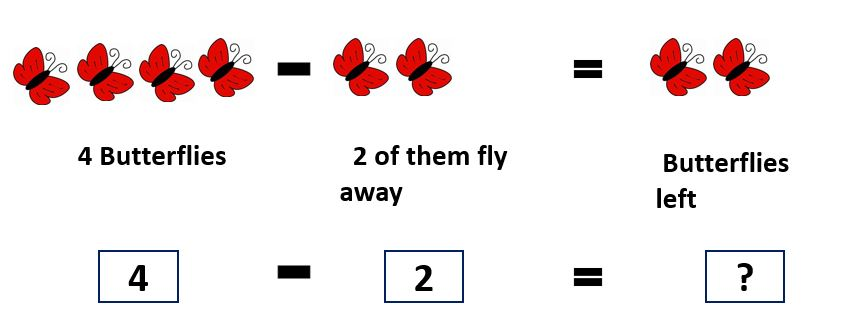a) 2
b) 3

Q.2) ​Count the number of objects that are not crossed out and select the write answer?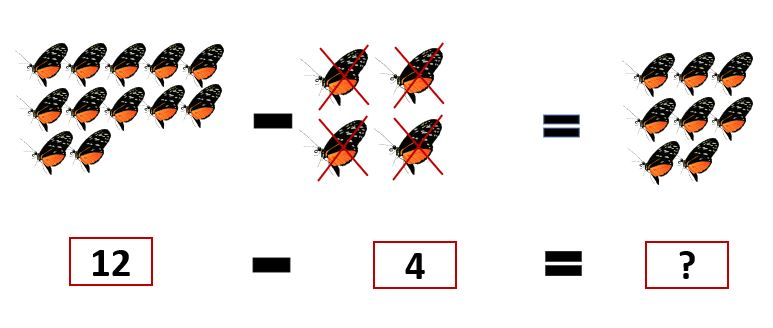a) 10
b) 8

Q.3) ​Subtract the following?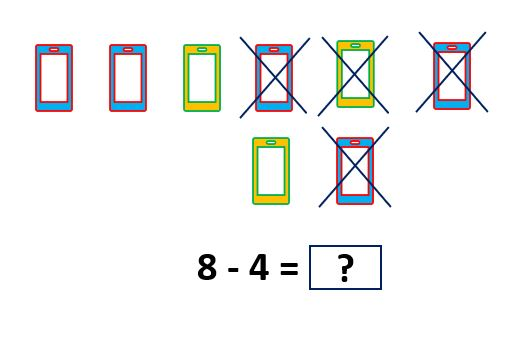a) 5
b) 4

​Q.4) ​​Fill in the missing box?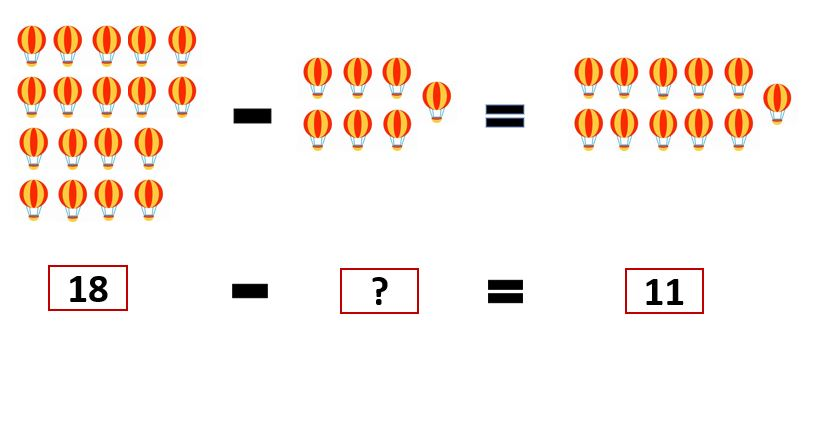a) 7
b) 9

Q.5) ​Fill in the missing box?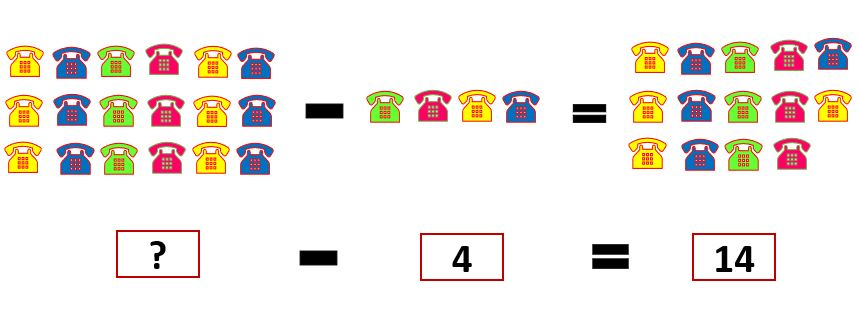a) 19
b) 18

Q.6) ​Choose the correct subtraction statement for the image?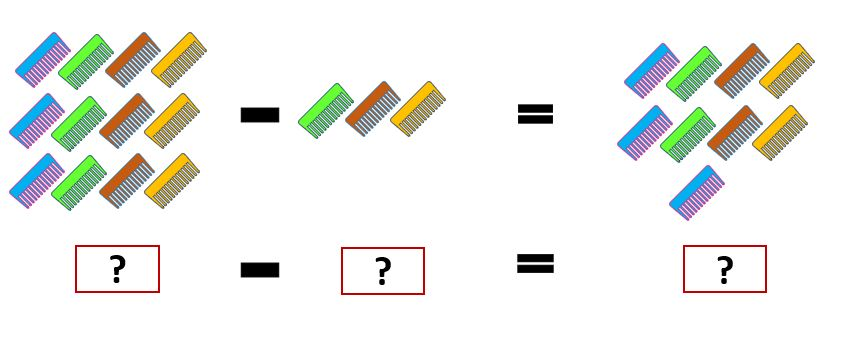a) ​12 – 5 = 7
b) ​12 – 3 = 9

Q.7) ​Subtract the Number using Number line?​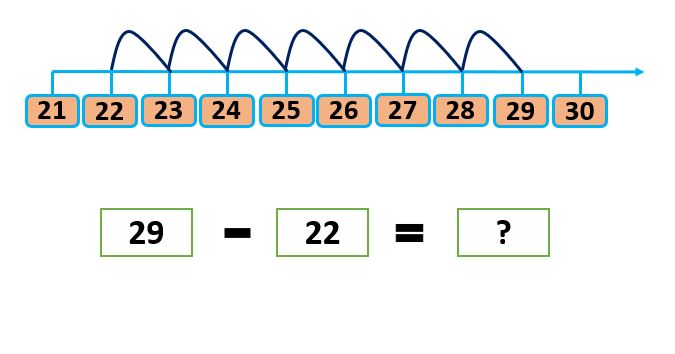a) 7
b) 9

Q.8) ​​Subtract the following numbers?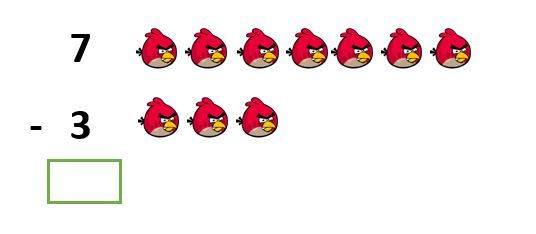a) 6
b) 4

​Q.9) ​Subtract the following numbers?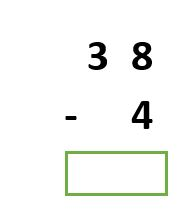a) 45
b) 34

​Q.10) ​​Choose the related subtraction facts for number 1 and all?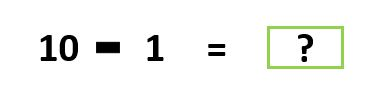a) 9
b) 7

​Q.11) ​Choose the related subtraction facts for number 2 and all?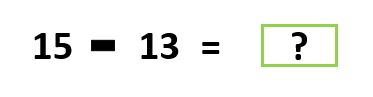a) 2
b) 6

​Q.12) ​​Subtract the following numbers?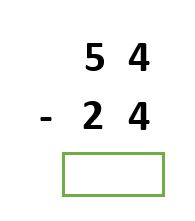a) 20
b) 30

​Q.13) ​Choose the related subtraction facts for number 3 and all?​​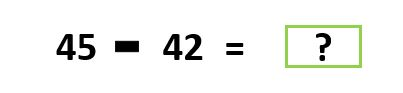a) 3
b) 7

​Q.14) Sumit had Rs.67. He gave Rs. 43 to her brother. How much money were left with him?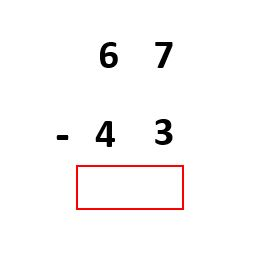a) 32
b) 24

Q.1) a. 2
Q.2) b. 8
Q.3) b. 4
Q.4) a. 7
Q.5) b. 18
Q.6) b. ​12 – 3 = 9
Q.7) a. 7
Q.8) b. 4
Q.9) b. 34
Q.10) a. 9
Q.11) a. 2
Q.12) b. 30
Q.13) a. 3
Q.14) b. 24